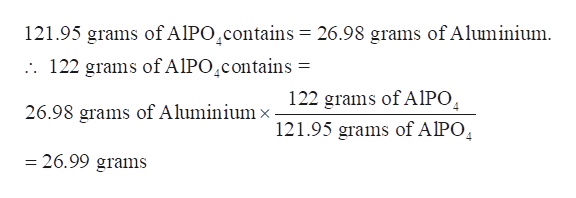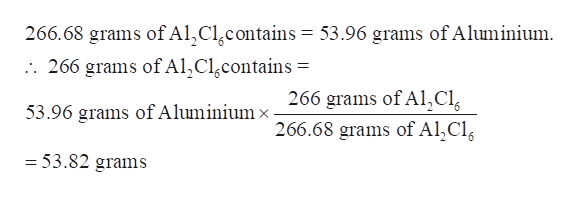# Determine which of the following contains the greatest mass of aluminum: 122g of AlPO4, 266g of Al2Cl6, or 225g of Al2S3.

Question
461 views

Determine which of the following contains the greatest mass of aluminum: 122g of AlPO4, 266g of Al2Cl6, or 225g of Al2S3.

check_circle

star
star
star
star
star
1 Rating
Step 1

We have to calculate mass of aluminium in 122g of AlPO4, 266g of Al2Cl6, and 225g of Al2S3 and then have to report which one has greatest mass of aluminium.

Step 2

Calculate the mass of Aluminium in 122g of AlPO4-

Molar mass of AlPO4 = 121.95 g/mol

As per the chemical formula, 1 mole of AlPO4 has 1 mole Al i.e. 26.98 grams. It means 121.95 grams of AlPO4 contains 26.98 grams of Aluminium.help_outlineImage Transcriptionclose121.95 grams of AIPO^contains = 26.98 grams of Aluminium 122 grams of AIPO contains = 122 grams of AIPO 121.95 grams of AIPO 26.98 grams of Aluminium x = 26.99 grams fullscreen
Step 3

Calculate the mass of Aluminium in 266g of Al2Cl6-

Molar mass of Al2Cl6 = 266.68 g/mol

As per the chemical formula, 1 mole of Al2Cl6 has 2 moles Al i.e. (2×26.98 ...help_outlineImage Transcriptionclose266.68 grams of Al,Clcontains = 53.96 grams of Aluminium 266 grams of Al,Clgcontains = 266 grams of Al,Cl 266.68 grams of Al,Cle 53.96 grams of Aluminium x = 53.82 grams fullscreen

#### Want to see this answer and more?

Solutions are written by subject experts who are available 24/7. Questions are typically answered within 1 hour.*

See Solution
*Response times may vary by subject and question.
Tagged in

### General Chemistry